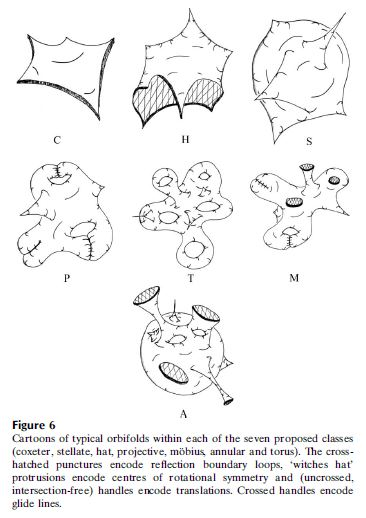Contents

# Contents

## Idea

An orbifold is much like a smooth manifold but possibly with singularities of the form of fixed points of group-actions.Where a smooth manifold is a space locally modeled on Cartesian spaces/Euclidean spaces $\mathbb{R}^n$, an orbifold is, more generally, a space that is locally modeled on smooth action groupoids (homotopy quotients) $\mathbb{R}^n\sslash G$ of a finite group $G$ acting on a Cartesian space.

graphics grabbed from Hyde-Ramsden-Robins 14

This turns out to be broadly captured(Moerdijk-Pronk 97, Moerdijk 02) by saying that an orbifold is a proper étale Lie groupoid. (Morita equivalent Lie groupoids correspond to the same orbifolds.)

The word orbifold was introduced in (Thurston 1992), while the original name was $V$-manifold (Satake), and was taken in a more restrictive sense, assuming that the actions of finite groups on the charts are always effective. Nowadays these are called effective orbifolds and those which are global quotients by a finite group are global quotient orbifolds.

There is also a notion of finite stabilizers in algebraic geometry. A singular variety is called an (algebraic) orbifold if it has only so-called orbifold singularities.

## Definition

An orbifold is a stack presented by an orbifold groupoid.

## Properties

### Global quotient orbifolds

In (ALR 07, theorem 1.23) is asserted that every effective orbifold $X$ (paracompact, Hausdorff) is isomorphic to a global quotient orbifold, specifically to a global quotient of $O(n)$ (where $n$ is the dimension of $X$) acting on the frame bundle of $X$ (which is a manifold).

### (Co)homology

It has been noticed that the topological invariants of the underlying topological space of an orbifold as a topological space with an orbifold structure are not appropriate, but have to be corrected leading to orbifold Euler characteristics, orbifold cohomology etc. One of the constructions which is useful in this respect is the inertia orbifold (the inertia stack of the original orbifold) which gives rise to “twisted sectors” in Hilbert space of a quantum field theory on the orbifold, and also to twisted sectors in the appropriate cohomology spaces. A further generalization gives multitwisted sectors.

## Examples

Orbifolds are in differential geometry what Deligne-Mumford stacks are in algebraic geometry. See also at geometric invariant theory and GIT-stable point.

If the finiteness condition is dropped one also speaks of orbispaces and generally of stacks.

Orbifolds may be regarded as a kind of stratified spaces.

Orbifolds in string theory:

### General

The concept originates in

• I. Satake, On a generalisation of the notion of manifold, Proc. Nat. Acad. Sci. U.S.A. 42 (1956), 359–363 (doi:10.1073/pnas.42.6.359)

• I. Satake, The Gauss–Bonnet theorem for $V$-manifolds, J. Math. Soc. Japan 9 (1957), 464–492.

• William Thurston, Three-dimensional geometry and topology, preliminary draft, University of Minnesota, Minnesota, (1992)

which in completed and revised form is available as his book:

The Geometry and Topology of Three-Manifolds; (web)

in particular the orbifold discussion is in chapter 13.

Survey of basic orbifold theory:

• Daryl Cooper, Craig Hodgson, Steve Kerckhoff, Three-dimensional Orbifolds and Cone-Manifolds, MSJ Memoirs Volume 5, 2000 (pdf, euclid:1389985812)

• Adam Kaye, Two-Dimensional Orbifolds, 2007 (pdf)

• Michael Davis, Lectures on orbifolds and reflection groups, 2008 (pdf)

• Joan Porti, An introduction to orbifolds, 2009 (pdf)

• Andrew Snowden, Introduction to orbifolds, 2011 (pdf)

• Alejandro Adem, Michele Klaus, Lectures on orbifolds and group cohomology (pdf, pdf)

• Francisco C. Caramello Jr, Introduction to orbifolds (arXiv:1909.08699)

Textbook account:

• Christian Lange, Orbifolds from a metric viewpoint (arXiv:1801.03472)

• Renato G. Bettiol, Andrzej Derdzinski, Paolo Piccione, Teichmüller theory and collapse of flat manifolds, Annali di Matematica (2018) 197: 1247 (arXiv:1705.08431, doi:10.1007/s10231-017-0723-7)

• S. T. Hyde, S. J. Ramsden and V. Robins, Unification and classification of two-dimensional crystalline patterns using orbifolds, Acta Cryst. (2014). A70, 319-337 (doi:10.1107/S205327331400549X)

Survey of applications in mathematical physics and notably in string theory:

Orbifolds often appear as moduli spaces in differential geometric setting:

The generalization of orbifolds to weighted branched manifolds is discussed in

• Dusa McDuff, Groupoids, branched manifolds and multisections, J. Symplectic Geom. Volume 4, Number 3 (2006), 259-315 (project euclid).

A reference dealing with the string topology of orbifolds is

• A. Adem, J. Leida and Y. Ruan, Orbifolds and Stringy Topology, Cambridge Tracts in Mathematics 171 (2007) (pdf)

### As Lie groupoids

Discussion of orbifold as Lie groupoids/differentiable stacks is in

Discussion of the corresponding perspective in algebraic geometry, via Deligne-Mumford stacks:

• Andrew Kresch, On the geometry of Deligne-Mumford stacks (doi:10.5167/uzh-21342, pdf), in: D. Abramovich, A. Bertram, L. Katzarkov, R. Pandharipande, M. Thaddeus (eds.) Algebraic Geometry: Seattle 2005, Proceedings of Symposia in Pure Mathematics 80, Providence, Rhode Island: American Mathematical Society 2009, 259-271 (pspum-80-1)

The mapping stacks of orbifolds are discussed in

An expected relation of orbifolds to global equivariant homotopy theory is discussed in

### As diffeological spaces

Discussion of orbifolds regarded as naive local quotient diffeological spaces:

### Orbifold cobordism

Orbifold cobordisms are discussed in

• K. S. Druschel, Oriented Orbifold Cobordism, Pacific J. Math., 164(2) (1994), 299-319.

• K. S. Druschel, The Cobordism of Oriented Three Dimensional Orbifolds, Pacific J. Math., bf 193(1) (2000), 45-55.

• Andres Angel, Orbifold cobordism (pdf)

### In string theory

In perturbative string theory, orbifolds as target spaces for a string sigma-model were first considered in

and then further developed notably in

And discussion of blow-up of orbifold singularities in string theory:

For orbifolds in string theory also the references at

Review of orbifolds in the context of string KK-compactifications and intersecting D-brane models includes

and for orbifolds of G2-manifolds for M-theory on G2-manifolds

For topological strings the path integral as a pull-push transform for target orbifolds – in analogy to what Gromov-Witten theory is for Deligne-Mumford stacks – has first been considered in

category: Lie theory